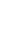2019-01-17 13:33

一、口算(我们的目标是：最终达到每分钟最低10道!)

98-70= 22+7= 50+26= 31-8= 62+8=

45-3= 70-5= 32+30= 46+4= 32-20=

38-5= 29+3= 23+4= 58-40= 9+63=

47-4= 36-3= 90-6= 87+8= 84-40=

43+30= 7+78= 58-7= 46-8= 8+61=

6+49= 36-7= 36-5= 43+8= 89-8=

30+42= 2+74= 63-20= 65+5= 75-9=

70-4= 5+36= 46+6= 62-3= 85-7=

共计( )分 共计( )分

二、解决问题

1、学校图书室有60本故事书，借出40本，还剩多少本?

=

2、果园里有27棵桃树，9棵梨树，桃树比梨树多多少棵?

=

3、妈妈有83元钱，买书用去30元，还剩多少元钱?

=

4、乐乐折了35个飞机，花花折了20个，花花比乐乐少折几个飞机?

=•2020年六年级英语测试题及答案5972次下载 点击下载
•一年级下册语文预习：看拼音写词语5821次下载 点击下载
•语文修辞手法作用大全及常见修辞手法4968次下载 点击下载
•五年级语文阅读习题推荐：张骞出使西域2858次下载 点击下载
•2020小学语文修辞手法练习题及答案7591次下载 点击下载
•2020年小学一年级下册英语练习册6385次下载 点击下载300次下载• 小学资讯
• 综合辅导
• 家庭教育
• 兴趣爱好

新东方小学辅导专区

 班级名称 上课地点 上课时间 费用 详细

焦点推荐

版权及免责声明

凡本网注明"稿件来源：新东方"的所有文字、图片和音视频稿件，版权均属新东方教育科技集团（含本网和新东方网） 所有，任何媒体、网站或个人未经本网协议授权不得转载、链接、转贴或以其他任何方式复制、发表。已经本网协议授权的媒体、网站，在下载使用时必须注明"稿件来源：新东方"，违者本网将依法追究法律责任。

本网未注明"稿件来源：新东方"的文/图等稿件均为转载稿，本网转载仅基于传递更多信息之目的，并不意味着赞同转载稿的观点或证实其内容的真实性。如其他媒体、网站或个人从本网下载使用，必须保留本网注明的"稿件来源"，并自负版权等法律责任。如擅自篡改为"稿件来源：新东方"，本网将依法追究法律责任。

如本网转载稿涉及版权等问题，请作者见稿后在两周内速来电与新东方网联系，电话：010-60908555。

阅读排行榜

学科提分辅导

家庭教育